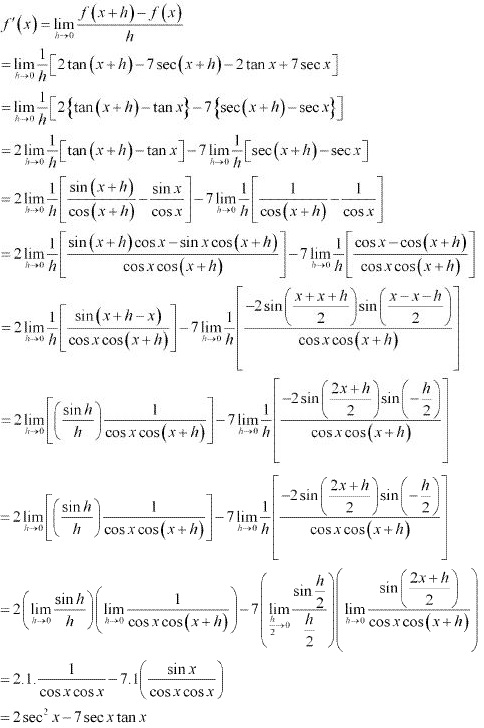# Class 11 Maths NCERT Solutions for Chapter 13 Limits and Derivatives Exercise 13.2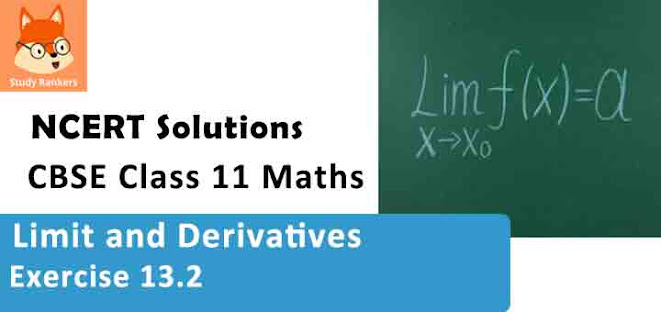### Limits and Derivatives Exercise 13.2 Solutions

1. Find the derivative of x2 – 2 at x = 10.

Solution

Let f(x) = x2 – 2. Accordingly,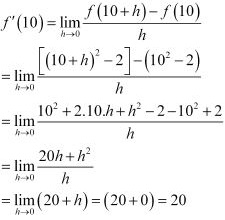Thus, the derivative of x2 – 2 at x = 10 is 20 .

2. Find the derivative of 99x at x = 100.

Solution

Let f(x) = 99x . Accordingly,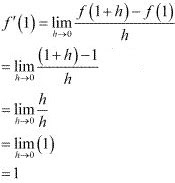Thus, the derivative of x at x = 1 is  1.

3. Find the derivative of x at x = 1.

Solution

Let f(x) = 99x, Accordingly,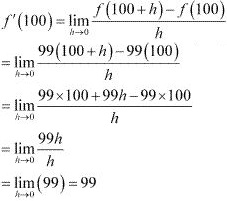Thus, the derivative of 99x at x = 100 is 99.

4. Find the derivative of the following functions from first principle.
(i) x3 – 27
(ii) (x – 1) (x – 2)
(iii) 1/x2
(iv) (x + 1)/(x - 1)

Solution

(i) Let f(x) = x3 - 27, Accordingly, from the first principle,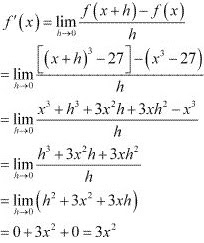(ii) Let f(x) = (x - 1)/(x - 2)  Accordingly, from the first principle,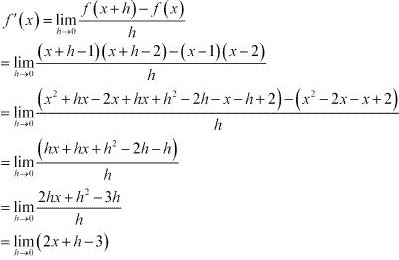= (2x + 0 - 3)
= 2x - 3

(iii) Let f(x) = 1/x2, Accordingly, from the first principle,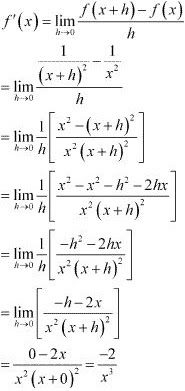(iv) let f(x) = (x + 1)/(x - 1). Accordingly, from the first principle,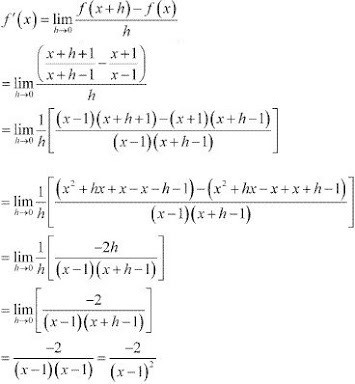5. For the function
f(x) = x100 /100 + x99 /99 + ....+ x2 /2 + x + 1
Prove that f '(1) = 100 f '(0)

Solution

The given function is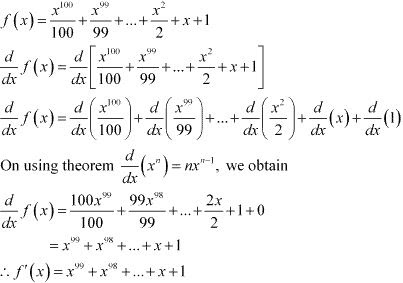At x = 0,
f '(0) = 1
At x = 1,
f '(1) = 199 + 198 + ... + 1 +1 = [1 + 1 +....+ 1 + 1] 100 terms  = 1 × 100 = 100
Thus, f '(1) = 100 × f1 (0)

6. Find the derivative of xn + axn-1 + a2 xn-2 + .....+ an-1 x + an for some fixed real number a

Solution

Let f(x) = xn + axn-1 + a2 xn-2 + ......+ an-1 x + an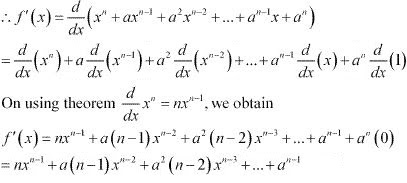7. For some constants a and b, find the derivative of
(i) (x – a) (x – b)
(ii) (ax2 + b)2
(iii) (x- a)/(x - b)

Solution

(i) Let f(x)  = (x - a)(x -b)
⇒ f(x) = x2 - (a +b)x + ab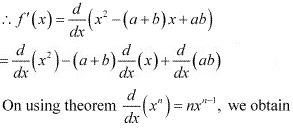f '(x) = 2x - (a + b) + 0 = 2x - a -b

(ii) Let f(x) = (ax2 + b2)
⇒ f(x) = a2 x4 + 2abx2 + b2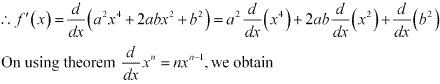f '(x) = a2(4x3) + 2ab (2x) + b2 (0)
= 4a2 x3 + 4abx
= 4ax(ax2 + b)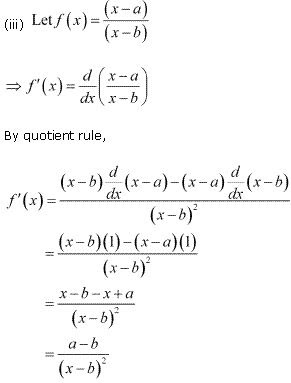8. Find the derivative of  (xn - an )/(x-a) for some constant a.

Solution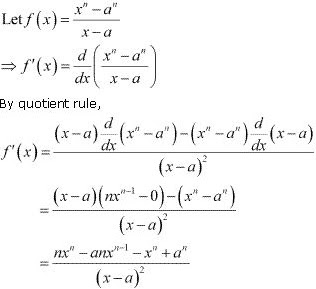9. Find the derivative of
(i) 2x - 3/4
(ii) (5x3 + 3x - 1)(x - 1)
(iii) x-3 (5 + 3x)
(iv) x5 (3 - 6x-9)
(v) x-4 (3 - 4x-5)
(vi) 2/(x + 1) - x2/(3x - 1)
Solution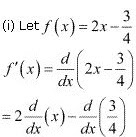= 2 - 0
= 2

(ii) Let f(x) = (5x3 + 3x - 1)(x- 1)
By Leibnitz product rule,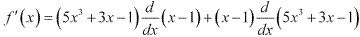= (5x3 + 3x - 1)(1) + (x - 1) (5.3x2 + 3 - 0)
- (5x3 + 3x - 1) + (x - 1)(15x3 + 3)
= 5x3 + 3x - 1 + 15x3 + 3x - 15x2 - 3
= 20x3 - 15x2 + 6x - 4

(iii) Let f(x) = x-3 (5 + 3x)
By Leibnitz product rule,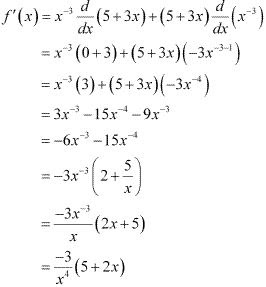(iv) Let f(x) = x5 (3 - 6x-9
By Leibnitz product rule,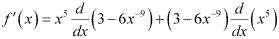= x5 [0 - 6(-9)x-9-1] + (3 - 6x-9)(5x4
= x5 (54x-10) + 15x4 - 30x-5
= 54x-5 + 15x4 - 30x-5
= 24x-5 + 15x4
= 15x4 + 24/x5

(v) Let f(x) = x-4 (3 - 4x-5
By Leibnitz product rule,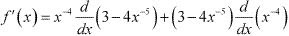= x-4 [0 - 4(-5)x-5-1] + (3 - 4x-5)(-4)x-4-1
= x-4 (20x-6)+ (3 -4x-5)(-4x-5
= 20x-10 - 12x-5 + 16x-10
= 36x-10 - 12x-5
= -12/x5 + 36/x10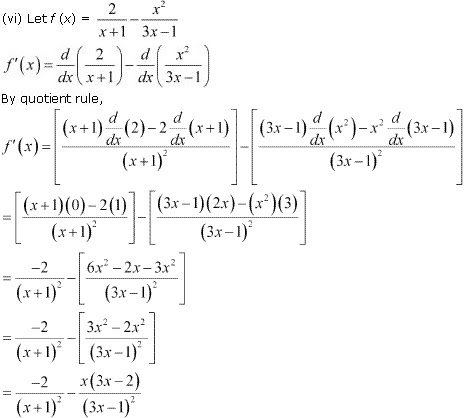10. Find the derivative of cos x from first principle.
Solution
Let f(x) = cos x, Accordingly, from the first principle,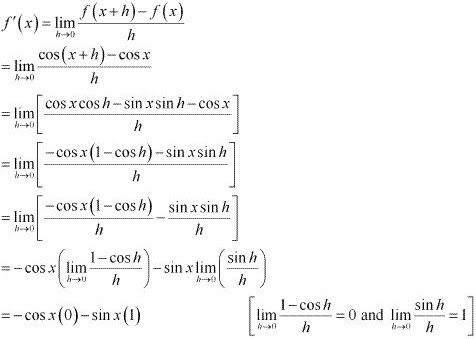= - sin x
∴ f '(x) = -sin x

11. Find the derivative of the following functions:
(i) sin x cos x
(ii) sec x
(iii) 5 sec x + 4 cos x
(iv) cosec x
(v) 3cot x + 5cosec x
(vi) 5sin x – 6cos x + 7
(vii) 2tan x – 7sec x
Solution
(i) Let f(x) = sin x cos x. Accordingly, from the first principle,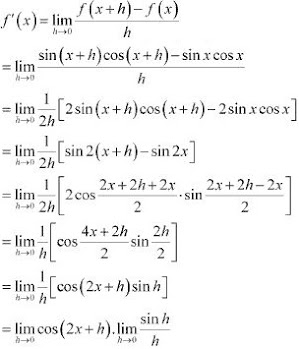= cos (2x + 0).1
= cos 2x

(ii) Let f(x) = sec x. Accordingly, from the first principle,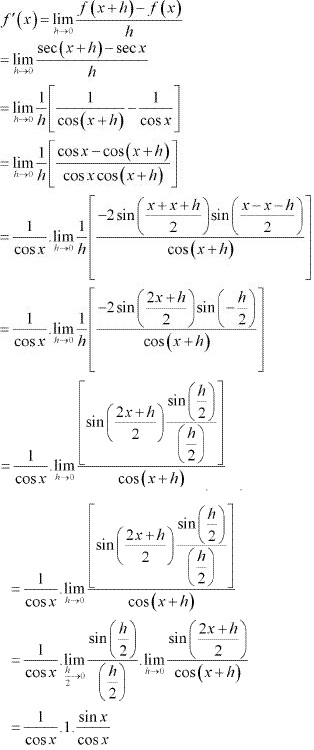= sec x tan x

(iii) Let f(x) = 5 sec x + 4cos x. Accordingly, from the first principle,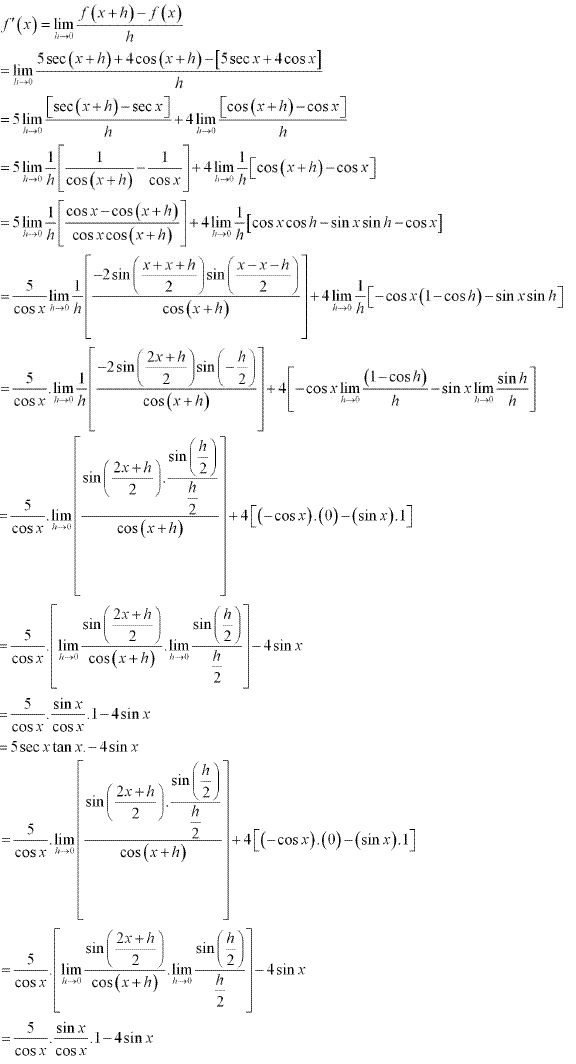(iv) Let f(x) = cosec x, Accordingly, from the first principle,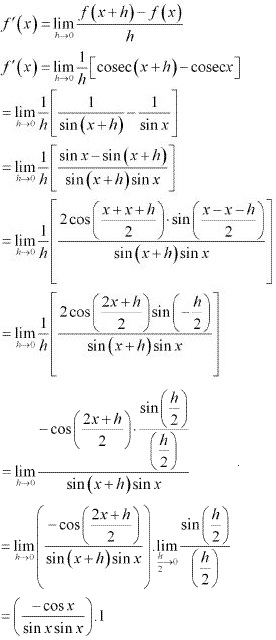(v) Let f(x) = 3cot x + 5cosec x. Accordingly, from the first principle,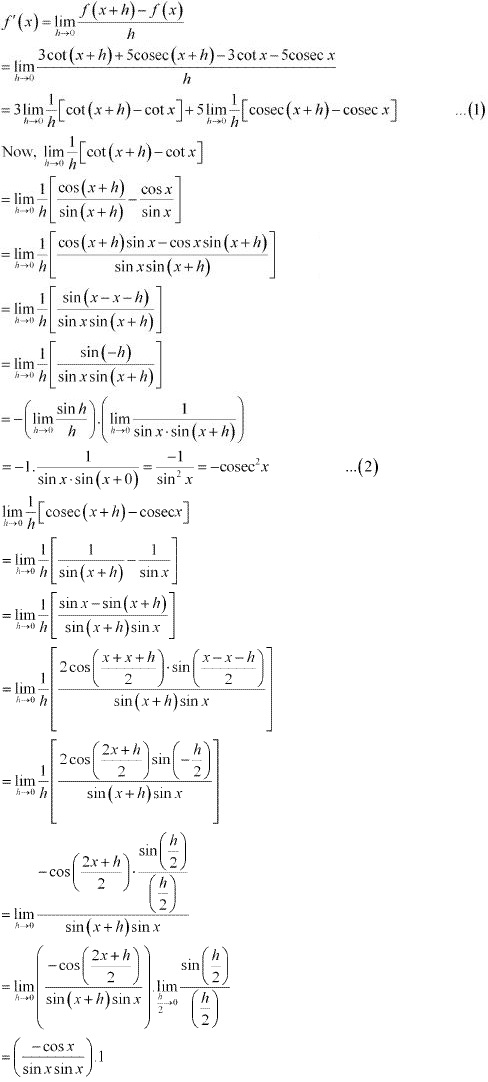= - cosecx cot x  ...(3)
From (1), (2) and (3), we obtain
f '(x) = -3cosec2 x - 5cosec x cot x

(vi) Let f(x) = 5sin x - 6 cosx + 7. Accordingly, from the first principle,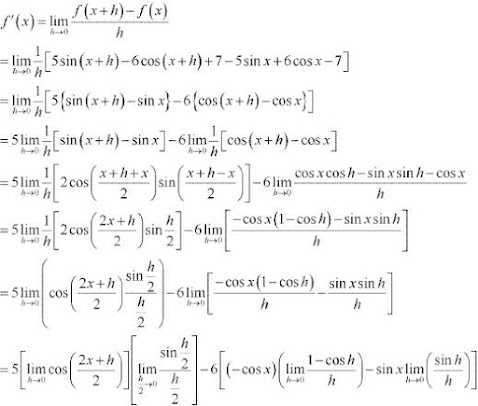= 5cosx. 1 -6[(-cos x).(0) - sinx. 1]
= 5 cos x + 6 sin x

(vii) Let f(x) = 2 tan x - 7 sec x. Accordingly, from the first principle,